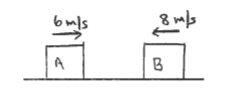Physics Practice Problems Elastic Collisions Practice Problems Solution: Blocks A and B are moving on a horizontal friction...

# Solution: Blocks A and B are moving on a horizontal frictionless air track. Block A has mass 3.0 kg and block B has mass 6.0 kg. Initially block A is moving to the right at 6.0 m/s and block B is moving to the left at 8.0 m/s. The blocks collide and after the collision block A is moving to the left at 4.0 m/s. What is the velocity of block B after the collision? A) 3.0 m/s to the left B) 3.0 m/s o the right C) 8.6 m/s to the left D) 8.6 m/s to the right E) 13.0 m/s to the left F) 13.0 m/s to the right G) None of the above answers

###### Problem

Blocks A and B are moving on a horizontal frictionless air track. Block A has mass 3.0 kg and block B has mass 6.0 kg. Initially block A is moving to the right at 6.0 m/s and block B is moving to the left at 8.0 m/s. The blocks collide and after the collision block A is moving to the left at 4.0 m/s. What is the velocity of block B after the collision?

A) 3.0 m/s to the left

B) 3.0 m/s o the right

C) 8.6 m/s to the left

D) 8.6 m/s to the right

E) 13.0 m/s to the left

F) 13.0 m/s to the right

G) None of the above answers